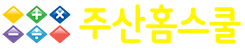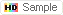#수강신청

### 강의보기

주산으로 시작하는 수학영재 만들기 프로그램!주산을 영어로...수강료 60,000원교재비 교재파일 제공강좌제공 기간 365일샘플보기강사 이수정강의시간과정유형 온라인

동영상
과목명
주산을 영어로... 60,000 원
교재명
 교재파일 무료제공
 In today's digital age, the human ability to think and numeracy paralyzed in mobile phone !  "We cannot continue to be like this. something has to be done!"=> After downloading, you can print it and use it as a textbook.Learning abacus makes you understand the concept of numbers and improves your mental arithmetic skills and basic arithmetic ability in a short period of time. Basically, math is limited to the area of the left brain responsible for logic languages. However, abacus arithmetic stimulates the visual, auditory and tactile without exception and actively helps the development of the right brain responsible for the artistic and sensory abilities as well as the left brain.This course covers basic operations like addition and subtraction using an abacus and makes you calculate easy, correct and fast.   Can learn abacus not boring with multimedia, graphics, subtitles, the motion of the character.Can calculate arithmetic operation easily and fun through the decomposition and combination of number.Adds a number and factor of an abacus to the animation of friends anthropomorphic abacus bead, stimulates curiosity and interest and approaches to learning with fun without resistance.Adds interest in math with animated storytelling.In parables and examples related to the number, it improves greatly mental arithmetic skills, calculation ability, logicality and thinking skills.The best means for learning the scientific understanding of mathematical concepts can be utilized for the abacus.Learn Addition and Subtraction using an Abacus The entire course leads you through the learning process step by step with multimedia teaching method of the new concept of combining unique character, animation and story telling and makes you study with ease and a lot of fun. It contains a number of tutorials, which are fundamental for you. There are many benefits to learning abacus calculation. You can have concentration, patience and sense in number including by working with the abacus and the brain develops while you enjoy the abacus. You can do the sum in your head without a calculator in mathematics. Also, anyone who wants to strengthen their understanding of abacus or anyone who wishes to learn from scratch or teach it can benefit from this course.A learning method that is very effective in improving the concentration that is scarce in the digital age (PC, mobile game) and developing brain such as mathematical thinking ability and creativity.Learn Basic Abacus Arithmetic quickly in this Course  - Simple Addition and Subtraction  - Mixed Addition and Subtraction  - Friend number of addition and subtraction for 5  - Complementary Numbers for 10  - 2 Digit Addition and Subtraction  - 3 Digit Addition and Subtraction  -  Multiplication and DivisionContents and Overview             Content is taught through interactive video lectures that include digital lecture with animated images and guided exercise problems. This course contains 78 lectures and 7 hours of content. I designed this abacus course to be easily understood by absolute beginners and for anyone who wishes to improve their calculation ability or wants to know how to teach. In this course, you will learn the Abacus Arithmetic, and you'll understand what it is and how to calculate numbers like addition and subtraction on the abacus. You'll learn, using the abacus, how to calculate several numbers in your head and finally, you'll have a remarkable ability in mathematics, concentration and agility moving your fingers on the abacus. By the end of this course, you'll have valuable skills that will help you quickly calculate without a calculator through the principle of the abacus. Even given any calculation problems, once you learn the abacus, the abacus is drawn to your head and it is possible to calculate faster. In this course, you'll find that you are able to solve math problems in addition and subtraction in your head and also mental arithmetic skills are improved.강의제목 강사 시간 강의내용
[맛보기]Addition & Subtraction 이수정 02분 15초
1- Get to know number and place on the abacus to try 이수정 04분 38초

Lesson 1

2- Addition from 1 to 4 이수정 03분 03초

Lesson 2

3- Exercise(9 Problems)  이수정 04분 04초
4- Subtraction from 1 to 4 이수정 04분 07초

Lesson 3

5- Exercise(6 Problems) 이수정 04분 09초
6- Mixed addition and subtraction up to 4(plus, minus 1.2.3.4) 이수정 03분 49초

Lesson 4

7- Exercise(6 Problems)  이수정 05분 58초
8- Addition from 5 to 7(plus 5,6,7) 이수정 04분 09초

Lesson 5

9- Exercise(8 Problems) 이수정 05분 38초
10- Addition from 8 to 9(plus 8,9) 이수정 03분 02초

Lesson 6

11- Exercise(6 Problems) 이수정 02분 41초
12- Subtraction the number to 9(from 1 to 5) 이수정 05분 22초

Lesson 7

13- Exercise(4 Problems) 이수정 02분 31초
14- Subtraction the number to 9(from 6 to 7) 이수정 04분 27초

Lesson 8

15- Exercise(8 Problems) 이수정 06분
16- Subtraction the number to 9(from 8 to 9) 이수정 04분 34초

Lesson 9

17- Exercise(6 Problems) 이수정 04분 49초
18- Mixed addition and subtraction up to 9 이수정 03분 47초

Lesson 10

19- Exercise(6 Problems) 이수정 03분 58초
20- Friend number of addition for 5(plus 1, 2) 이수정 04분 35초

Lesson 11

21- Exercise(6 Problems) 이수정 06분 02초
22- Friend number of addition for 5(plus 3, 4) 이수정 03분 16초

Lesson 12

23- Exercise(6 Problems) 이수정 05분 15초
24- Friend number of subtraction for 5(minus 1, 2) 이수정 04분 29초

Lesson 13

25- Exercise(5 Problems) 이수정 05분 34초
26- Friend number of subtraction for 5(minus 3, 4) 이수정 02분 39초

Lesson 14

27- Exercise(5 Problems) 이수정 04분 51초
28- Addition: Complementary numbers for 10(plus 1, 2) 이수정 03분 46초

Lesson 15

29- Exercise(5 Problems) 이수정 04분 56초
30- Addition: Complementary numbers for 10(plus 3, 4) 이수정 04분 31초

Lesson 16

31- Exercise(6 Problems) 이수정 05분 34초
32-Addition: Complementary numbers for 10(plus 5, 6) 이수정 04분 19초

Lesson 17

33- Exercise(5 Problems) 이수정 05분 21초
34- Addition: Complementary numbers for 10(plus 7, 8, 9) 이수정 06분 01초

Lesson 18

35- Exercise(5 Problems) 이수정 05분 28초
36- Subtraction: Complementary numbers for 10(minus 1, 2) 이수정 05분 11초

Lesson 19

37- Exercise(6 Problems) 이수정 06분 43초
38- Subtraction: Complementary numbers for 10(minus 3, 4) 이수정 05분 52초

Lesson 20

39- Exercise(5 Problems) 이수정 06분 57초
40- Subtraction: Complementary numbers for 10(minus 5, 6) 이수정 04분 35초

Lesson 21

41- Exercise(5 Problems) 이수정 06분 15초
42- Subtraction: Complementary numbers for 10(minus 7, 8, 9) 이수정 05분 05초

Lesson 22

43- Exercise(5 Problems) 이수정 06분 18초
44- Friend number of subtraction for 5(2)(minus 1, 2, 3, 4) 이수정 04분 40초

Lesson 23

45- Exercise(5 Problems) 이수정 05분 14초
46- Addition using 10&5(plus 6, 7, 8, 9)-Figures with the shape 이수정 04분 58초

Lesson 24

47- Exercise(7 Problems) 이수정 07분 58초
48- Subtraction using 10&5(minus 6,7,8,9,)-Figures with the shape 이수정 04분 54초

Lesson 25

49- Exercise(6 Problems) 이수정 07분 19초
50- Mixed calculation 2 digit &1 digit 이수정 06분 39초

Lesson 26

51- Exercise(5 Problems) 이수정 06분 24초
52- 2 digit addition(1) - (the answer is less than 50) 이수정 05분 42초

Lesson 27

53- Exercise(5 Problems) 이수정 06분 58초
54- 2 digit subtraction(1) - (the answer is less than 50) 이수정 04분 43초

Lesson 28

55- Exercise(5 Problems) 이수정 06분 48초
56- 2 digit addition(2) - (the answer is more than 50) 이수정 04분 18초

Lesson 29

57- Exercise(6 Problems) 이수정 09분 31초
58- 2 digit subtraction(2) - (the answer is more than 50) 이수정 05분 14초

Lesson 30

59- Exercise(5 Problems) 이수정 08분 06초
60- 2 digit addition(3) - (the answer is between 50 and 100) 이수정 05분 09초

Lesson 31

61- Exercise(6 Problems) 이수정 06분 07초
62- 2 digit subtraction(3) - (the answer is between 50 and 100) 이수정 03분 39초

Lesson 32

63- Exercise(6 Problems) 이수정 08분 45초
64- Double digit addition(the answer is more than 100) 이수정 08분 09초

Lesson 33

65- Exercise(6 Problems) 이수정 07분 23초
66- Double digit subtraction(the answer is near 100) 이수정 10분 22초

Lesson 34

67- Exercise(6 Problems) 이수정 09분 37초
68- Addition & subtraction of 5 rows(1) Exercise(6 Problems) 이수정 14분 21초

Lesson 35

69- Addition & subtraction of 5 rows(2) Exercise(6 Problems) 이수정 13분 33초

Lesson 36

70- 3 digit addition(1) 이수정 05분 12초

Lesson 37

71- Exercise(5 Problems) 이수정 06분 40초
72- 3 digit subtraction(1) 이수정 05분 46초

Lesson 38

73- Exercise(6 Problems) 이수정 07분 12초
74- 3 digit addition(2) 이수정 05분 14초

Lesson 39

75- Exercise(5 Problems) 이수정 10분 13초
76- 3 digit subtraction(2) 이수정 04분 45초

Lesson 40

77- Exercise(5 Problems) 이수정 11분 52초